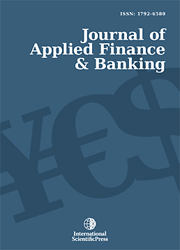# Journal of Applied Finance & Banking

#### The Amendment and Empirical Test of Arbitrage Pricing Models

•[ Download ]
• Abstact

The classical APT model is of the form  rj E(rj) = βj(I EI ) +εj , where  rj E(rj)  is the earning deviation (called basic variance-profit) of the security j, I is a common factor. This paper considers the impact on the securities return caused by the skewness and kurtosis of the stock returns distributions, and poses a re-modified the arbitrage pricing model as follows  rj= E(rj)  + βj(I EI ) +θj(I EI )^2 +λj(I EI )^3 +δj(I EI )^4 +εj

Based on the regression analysis method, and the fitting degree, one can arrive at this re-modified model has a more reasonable explanation level for securities pricing.ISSN: 1792-6599 (Online)
1792-6580 (Print)